# Excel

The Nordic Seas Excel loudspeaker drivers are absolute top components regarding innovation and quality. They are usually found in finished loudspeakers of the upper price segment. We are offering quite a few kits like Thor, Ex Trio 22, ExMotion, ExMotion 04, Odin and EX1-3.

## Tweeter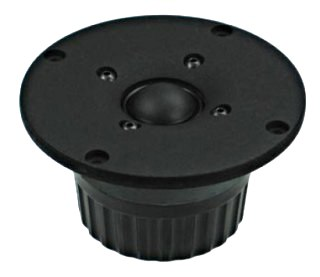## Excel T25CF001 (E0006-06)

order no. seas-E0006-06

EUR 135,50excl. VAT: € 116.81 / \$ 137.84

26 mm dome tweeter with fabric dome. The speaker has a 6 mm solid metal front plate. A double magnet system increases sensitivity and provides better control of the voice coil. It reduces the magnetic stray fields, what is good for A/V applications.

• power handling (continuous/programme) = 90/200(>2500Hz/12dB) W
• frequency range = 2000-25000 Hz
• resonance frequency fs = 700 Hz
• impedance R = 6 Ohm
• sound pressure level SPL = 91 dB (2,83V; 1m)
• DC resistance Re = 4,6 Ohm
• force factor BL = 3,5 N/A
• voice coil inductance L = 0,05 mH
• effective piston radiating area Sd = 7 cm2
• effective mechanical mass incl. air load mms = 0,33 g
• voice coil diameter = 26 mm
• maximum peak linear excursion vibration xlin = +/- 0,25 mm
• mounting diameter d = 85 mm
• overall diameter d = 110 mm
• mounting depth (not countersunk) t = 53 mm
PDF data sheet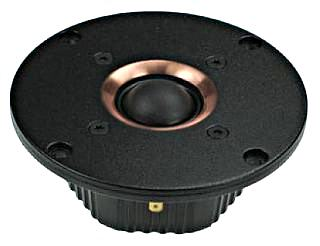## Excel T25CF002 (E0011-06)

order no. seas-E0011-06

EUR 322,66excl. VAT: € 278.16 / \$ 328.22

26 mm dome tweeter with fabric dome. The speaker has a 6 mm solid metal front plate. A unique magnet system based on 6 radially magnetized Neodymium magnet blocks is used.

• power handling (continuous/programme) = 90/200(>2500Hz/12dB) W
• frequency range = 2000-25000 Hz
• resonance frequency fs = 500 Hz
• impedance R = 6 Ohm
• sound pressure level SPL = 89 dB (2,83V; 1m)
• DC resistance Re = 4,6 Ohm
• force factor BL = 3,1 N/A
• voice coil inductance L = 0,05 mH
• effective piston radiating area Sd = 7 cm2
• effective mechanical mass incl. air load mms = 0,37 g
• voice coil diameter = 26 mm
• maximum peak linear excursion vibration xlin = +/- 0,5 mm
• mounting diameter d = 75 mm
• overall diameter d = 110 mm
• mounting depth (not countersunk) t = 41 mm
PDF data sheet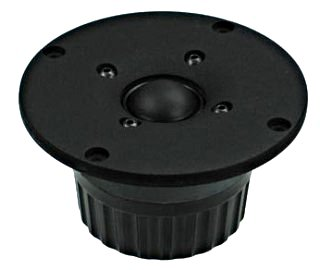## Excel T25C003 (E0036-06)

order no. seas-E0036-06

26 mm dome tweeter with fabric dome. The speaker has a 6 mm solid metal front plate. A double magnet system increases sensitivity and provides better control of the voice coil. It reduces the magnetic stray fields, what is good for A/V applications.

• power handling (continuous/programme) = 90/200(>2500Hz/12dB) W
• frequency range = 2000-25000 Hz
• resonance frequency fs = 600 Hz
• impedance R = 6 Ohm
• sound pressure level SPL = 91 dB (2,83V; 1m)
• DC resistance Re = 4,6 Ohm
• force factor BL = 3,5 N/A
• voice coil inductance L = 0,05 mH
• effective piston radiating area Sd = 7 cm2
• effective mechanical mass incl. air load mms = 0,33 g
• voice coil diameter = 26 mm
• maximum peak linear excursion vibration xlin = +/- 0,25 mm
• mounting diameter d = 73 mm
• overall diameter d = 110 mm
• mounting depth (not countersunk) t = 59 mm
PDF data sheet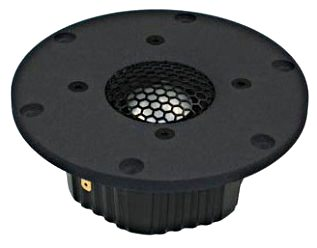## Excel T29AF001 (E0039)

order no. seas-E0039-06

26 mm dome tweeter with aluminium dome. The speaker has a 6 mm solid metal front plate. A unique magnet system based on 6 radially magnetized Neodymium magnet blocks is used.

• power handling (continuous/programme) = 100/220(>2500Hz/12dB) W
• frequency range = 2000-22000 Hz
• resonance frequency fs = 500 Hz
• impedance R = 6 Ohm
• sound pressure level SPL = 92 dB (2,83V; 1m)
• DC resistance Re = 4,7 Ohm
• force factor BL = 3,9 N/A
• voice coil inductance L = 0,05 mH
• effective piston radiating area Sd = 8 cm2
• effective mechanical mass incl. air load mms = 0,39 g
• voice coil diameter = 26 mm
• maximum peak linear excursion vibration xlin = +/- 0,5 mm
• mounting diameter d = 85 mm
• overall diameter d = 110 mm
• mounting depth (not countersunk) t = 43 mm
PDF data sheet

## Excel T29CF001 (E0038-06)

order no. seas-E0038-06

26 mm dome tweeter with fabric dome. The speaker has a 6 mm solid metal front plate. A unique magnet system based on 6 radially magnetized Neodymium magnet blocks is used.

• power handling (continuous/programme) = 90/200(>2500Hz/12dB) W
• frequency range = 2000-25002 Hz
• resonance frequency fs = 550 Hz
• impedance R = 6 Ohm
• sound pressure level SPL = 93 dB (2,83V; 1m)
• DC resistance Re = 4,7 Ohm
• force factor BL = 3,9 N/A
• voice coil inductance L = 0,05 mH
• effective piston radiating area Sd = 8 cm2
• effective mechanical mass incl. air load mms = 0,35 g
• voice coil diameter = 26 mm
• maximum peak linear excursion vibration xlin = +/- 0,5 mm
• mounting diameter d = 85 mm
• overall diameter d = 110 mm
• mounting depth (not countersunk) t = 43 mm
PDF data sheet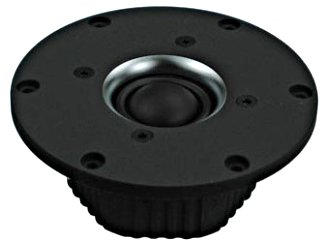## Excel T29CF002 (E0040-06) Crescendo

order no. seas-E0040-06

EUR 378,22excl. VAT: € 326.05 / \$ 384.74

26 mm dome tweeter with fabric dome. The speaker has a 6 mm solid metal front plate. A unique magnet system based on 6 radially magnetized Neodymium magnet blocks is used.

• power handling (continuous/programme) = 90/200 W
• frequency range = 2000-25000 Hz
• resonance frequency fs = 500 Hz
• impedance R = 6 Ohm
• sound pressure level SPL = 92,5 dB (2,83V; 1m)
• DC resistance Re = 4,7 Ohm
• force factor BL = 3,5 N/A
• voice coil inductance L = 0,05 mH
• effective piston radiating area Sd = 8 cm2
• effective mechanical mass incl. air load mms = 0,35 g
• voice coil diameter = 26 mm
• maximum peak linear excursion vibration xlin = +/- 0,5 mm
• mounting diameter d = 85 mm
• overall diameter d = 110 mm
• mounting depth (not countersunk) t = 43 mm
PDF data sheet

## Excel T29B001 (E0058-06) Beryllium

order no. seas-E0058-06

EUR 1880,37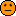excl. VAT: € 1621.01 / \$ 1912.79

26 mm dome tweeter with beryllium dome. The speaker has a 6 mm solid metal front plate. The magnet system is based on Neodymium technology.

• power handling (continuous/programme) = 90/200 W
• frequency range = 2000-25000 Hz
• resonance frequency fs = 533 Hz
• impedance R = 6 Ohm
• sound pressure level SPL = 93,6 dB (2,83V; 1m)
• DC resistance Re = 4,6 Ohm
• force factor BL = 3,5 N/A
• voice coil inductance L = 0,01 mH
• effective piston radiating area Sd = 8 cm2
• effective mechanical mass incl. air load mms = 0,3 g
• voice coil diameter = 26 mm
• maximum peak linear excursion vibration xlin = +/- 0,25 mm
• mounting diameter d = 85,5 mm
• overall diameter d = 110 mm
• mounting depth (not countersunk) t = 30 mm
PDF data sheet

## Excel T29D001 (E0100-04) Diamond

order no. seas-E0100-04

EUR 7401,58excl. VAT: € 6380.67 / \$ 7529.19

26 mm dome tweeter with high qualitydi dome. The speaker has a 4 mm solid metal front plate. The magnet system is based on Neodymium technology.

• power handling (continuous/programme) = 55/150 W
• frequency range = 1800-50000 Hz
• resonance frequency fs = 530 Hz
• impedance R = 4 Ohm
• sound pressure level SPL = 91 dB (2,83V; 1m)
• DC resistance Re = 3,8 Ohm
• force factor BL = 2,5 N/A
• voice coil inductance L = 0,01 mH
• effective piston radiating area Sd = 8 cm2
• effective mechanical mass incl. air load mms = 0,36 g
• voice coil diameter = 26 mm
• maximum peak linear excursion vibration xlin = +/- 0,7 mm
• mounting diameter d = 85 mm
• overall diameter d = 100 mm
• mounting depth (not countersunk) t = 33 mm
PDF data sheet

## Excel T35C002 (E0055-06)

order no. seas-E0055-06

EUR 262,80excl. VAT: € 226.55 / \$ 267.33

35 mm dome tweeter with fabric dome. The speaker has a 6 mm solid metal front plate.

• power handling (continuous/programme) = 100/250 W
• frequency range = 1500-25000 Hz
• resonance frequency fs = 620 Hz
• impedance R = 6 Ohm
• sound pressure level SPL = 95 dB (2,83V; 1m)
• DC resistance Re = 4,6 Ohm
• force factor BL = 4,3 N/A
• voice coil inductance L = 0,06 mH
• effective piston radiating area Sd = 11,9 cm2
• effective mechanical mass incl. air load mms = 0,47 g
• voice coil diameter = 35 mm
• maximum peak linear excursion vibration xlin = +/- 0,5 mm
• mounting diameter d = 85,5 mm
• overall diameter d = 110 mm
• mounting depth (not countersunk) t = 30 mm
PDF data sheet

## Woofer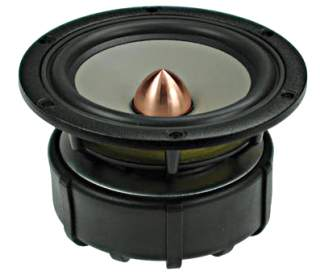recommended cabinet 1:
closed cabinet with 1,3 L volume
from 100/63 Hz (-3dB/-8dB)

recommended cabinet 2:
4,4 L volume bass reflex cabinet
with HP35 reflex tube, 21 cm long.
from 52/41 Hz (-3dB/-8dB).

## Excel W12CY001 (E0021-08S)

order no. seas-E0021-08S

EUR 274,89excl. VAT: € 236.97 / \$ 279.63

11 cm bass driver with magnesium cone.

• power handling (continuous/programme) = 70/200 W
• frequency range = 60-3500 Hz
• resonance frequency fs = 45 Hz
• impedance R = 8 Ohm
• sound pressure level SPL = 84 dB (2,83V; 1m)
• DC resistance Re = 5,6 Ohm
• force factor BL = 5,4 N/A
• voice coil inductance L = 0,43 mH
• effective piston radiating area Sd = 50 cm2
• effective mechanical mass incl. air load mms = 7,5 g
• equivalent volume of compliance Vas = 6 l
• total Q factor Qts = 0,36 (Qms=2,5, Qes=0,42)
• voice coil diameter = 26 mm
• maximum peak linear excursion vibration xlin = +/- 3 mm
• mounting diameter d = 99 mm
• overall diameter d = 120,4 mm
• mounting depth (not countersunk) t = 66,5 mm
PDF data sheet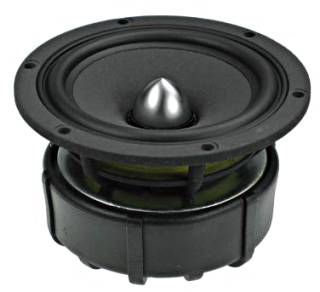recommended cabinet 1:
closed cabinet with 0,9 L volume
from 130/82 Hz (-3dB/-8dB)

recommended cabinet 2:
3,2 L volume bass reflex cabinet
with HP35 reflex tube, 16 cm long.
from 66/53 Hz (-3dB/-8dB).

## Excel W12CY003 (E0044-08S)

order no. seas-E0044-08S

EUR 223,23excl. VAT: € 192.44 / \$ 227.08

11 cm bass driver with paper cone.

• power handling (continuous/programme) = 70/200 W
• frequency range = 60-3500 Hz
• resonance frequency fs = 60 Hz
• impedance R = 8 Ohm
• sound pressure level SPL = 85,5 dB (2,83V; 1m)
• DC resistance Re = 5,5 Ohm
• force factor BL = 5,5 N/A
• voice coil inductance L = 0,41 mH
• effective piston radiating area Sd = 50 cm2
• effective mechanical mass incl. air load mms = 6,1 g
• equivalent volume of compliance Vas = 4 l
• total Q factor Qts = 0,37 (Qms=2,3, Qes=0,44)
• voice coil diameter = 26 mm
• maximum peak linear excursion vibration xlin = +/- 3 mm
• mounting diameter d = 99 mm
• overall diameter d = 120,4 mm
• mounting depth (not countersunk) t = 66,5 mm
PDF data sheet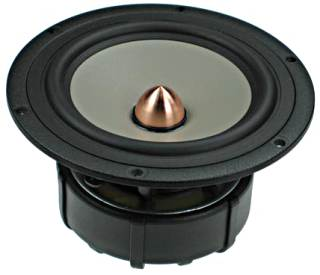recommended cabinet 1:
closed cabinet with 3 L volume
from 85/53 Hz (-3dB/-8dB)

recommended cabinet 2:
10,2 L volume bass reflex cabinet
with HP35 reflex tube, 11 cm long.
from 44/34 Hz (-3dB/-8dB).

## Excel W15CY001 (E0015-08S)

order no. seas-E0015-08S

EUR 250,52excl. VAT: € 215.97 / \$ 254.84

13 cm bass driver with magnesium cone.

• power handling (continuous/programme) = 70/200 W
• frequency range = 50-3000 Hz
• resonance frequency fs = 38 Hz
• impedance R = 8 Ohm
• sound pressure level SPL = 85,5 dB (2,83V; 1m)
• DC resistance Re = 5,5 Ohm
• force factor BL = 5,5 N/A
• voice coil inductance L = 0,49 mH
• effective piston radiating area Sd = 75 cm2
• effective mechanical mass incl. air load mms = 9,5 g
• equivalent volume of compliance Vas = 14 l
• total Q factor Qts = 0,36 (Qms=2,22, Qes=0,43)
• voice coil diameter = 26 mm
• maximum peak linear excursion vibration xlin = +/- 4 mm
• mounting diameter d = 112 mm
• overall diameter d = 146 mm
• mounting depth (not countersunk) t = 75,5 mm
PDF data sheet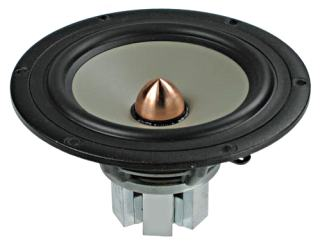recommended cabinet 1:
closed cabinet with 4,4 L volume
from 73/46 Hz (-3dB/-8dB)

recommended cabinet 2:
17 L volume bass reflex cabinet
with HP50 reflex tube, 20 cm long.
from 36/29 Hz (-3dB/-8dB).

## Excel W15CH001 (E0037-08S)

order no. seas-E0037-08S

EUR 329,48excl. VAT: € 284.03 / \$ 335.16

13 cm bass driver with magnesium cone. A unique magnet system based on 6 radially magnetized Neodymium magnet blocks is used.

• power handling (continuous/programme) = 70/200 W
• frequency range = 50-3000 Hz
• resonance frequency fs = 38 Hz
• impedance R = 8 Ohm
• sound pressure level SPL = 85 dB (2,83V; 1m)
• DC resistance Re = 5,5 Ohm
• force factor BL = 5,1 N/A
• voice coil inductance L = 0,49 mH
• effective piston radiating area Sd = 75 cm2
• effective mechanical mass incl. air load mms = 9,5 g
• equivalent volume of compliance Vas = 14 l
• total Q factor Qts = 0,42 (Qms=2,58, Qes=0,5)
• voice coil diameter = 26 mm
• maximum peak linear excursion vibration xlin = +/- 4 mm
• mounting diameter d = 112 mm
• overall diameter d = 146 mm
• mounting depth (not countersunk) t = 76 mm
PDF data sheet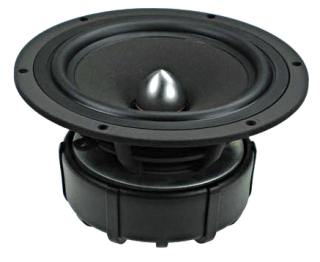recommended cabinet 1:
closed cabinet with 4,5 L volume
from 88/55 Hz (-3dB/-8dB)

recommended cabinet 2:
18 L volume bass reflex cabinet
with HP50 reflex tube, 11 cm long.
from 42/35 Hz (-3dB/-8dB).

## Excel W15LY001 (E0041-08S)

order no. seas-E0041-08S

EUR 218,35excl. VAT: € 188.23 / \$ 222.11

13 cm bass driver with paper cone.

• power handling (continuous/programme) = 60/250 W
• frequency range = 50-4000 Hz
• resonance frequency fs = 49 Hz
• impedance R = 8 Ohm
• sound pressure level SPL = 86,5 dB (2,83V; 1m)
• DC resistance Re = 5,7 Ohm
• force factor BL = 4,6 N/A
• voice coil inductance L = 0,3 mH
• effective piston radiating area Sd = 75 cm2
• effective mechanical mass incl. air load mms = 6,28 g
• equivalent volume of compliance Vas = 12 l
• total Q factor Qts = 0,45 (Qms=2,21, Qes=0,56)
• voice coil diameter = 26 mm
• maximum peak linear excursion vibration xlin = +/- 5 mm
• mounting diameter d = 112 mm
• overall diameter d = 146 mm
• mounting depth (not countersunk) t = 75,5 mm
PDF data sheet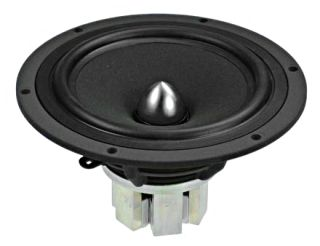recommended cabinet 1:
closed cabinet with 1,6 L volume
from 135/85 Hz (-3dB/-8dB)

recommended cabinet 2:
6,2 L volume bass reflex cabinet
with HP50 reflex tube, 15 cm long.
from 67/54 Hz (-3dB/-8dB).

## Excel M15CH002 (E0043-06S)

order no. seas-E0043-06S

EUR 281,71excl. VAT: € 242.85 / \$ 286.57

13 cm bass-midrange driver with paper cone. A unique magnet system based on 6 radially magnetized Neodymium magnet blocks is used.

• power handling (continuous/programme) = 70/200 W
• frequency range = 60-3500 Hz
• resonance frequency fs = 67 Hz
• impedance R = 6 Ohm
• sound pressure level SPL = 88,5 dB (2,83V; 1m)
• DC resistance Re = 4,9 Ohm
• force factor BL = 5,3 N/A
• voice coil inductance L = 0,43 mH
• effective piston radiating area Sd = 75 cm2
• effective mechanical mass incl. air load mms = 6,75 g
• equivalent volume of compliance Vas = 6 l
• total Q factor Qts = 0,4 (Qms=1,7, Qes=0,52)
• voice coil diameter = 26 mm
• maximum peak linear excursion vibration xlin = +/- 3 mm
• mounting diameter d = 112 mm
• overall diameter d = 146 mm
• mounting depth (not countersunk) t = 76 mm
PDF data sheet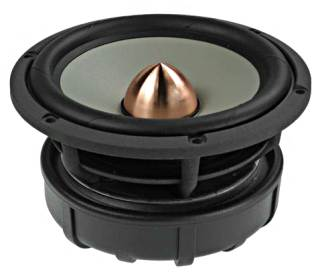recommended cabinet 1:
closed cabinet with 2,8 L volume
from 87/55 Hz (-3dB/-8dB)

recommended cabinet 2:
9,1 L volume bass reflex cabinet
with HP35 reflex tube, 12 cm long.
from 46/36 Hz (-3dB/-8dB).

## Excel W16NX001 (E0049-04S)

order no. seas-E0049-04S

EUR 338,25excl. VAT: € 291.59 / \$ 344.08

13 cm bass driver with magnesium cone.

• power handling (continuous/programme) = 80/250 W
• frequency range = 45-2500 Hz
• resonance frequency fs = 37 Hz
• impedance R = 4 Ohm
• sound pressure level SPL = 88 dB (2,83V; 1m)
• DC resistance Re = 3 Ohm
• force factor BL = 5,3 N/A
• voice coil inductance L = 0,25 mH
• effective piston radiating area Sd = 94 cm2
• effective mechanical mass incl. air load mms = 14,8 g
• equivalent volume of compliance Vas = 15 l
• total Q factor Qts = 0,33 (Qms=2,66, Qes=0,38)
• voice coil diameter = 39 mm
• maximum peak linear excursion vibration xlin = +/- 7 mm
• mounting diameter d = 126 mm
• overall diameter d = 146 mm
• mounting depth (not countersunk) t = 73,5 mm
PDF data sheetrecommended cabinet 1:
closed cabinet with 2,8 L volume
from 89/56 Hz (-3dB/-8dB)

recommended cabinet 2:
9,3 L volume bass reflex cabinet
with HP35 reflex tube, 11 cm long.
from 47/37 Hz (-3dB/-8dB).

## Excel W16NX001 (E0049-08S)

order no. seas-E0049-08S

EUR 334,35excl. VAT: € 288.23 / \$ 340.11

13 cm bass driver with magnesium cone.

• power handling (continuous/programme) = 80/250 W
• frequency range = 45-2500 Hz
• resonance frequency fs = 39 Hz
• impedance R = 8 Ohm
• sound pressure level SPL = 85 dB (2,83V; 1m)
• DC resistance Re = 6,2 Ohm
• force factor BL = 7,3 N/A
• voice coil inductance L = 0,41 mH
• effective piston radiating area Sd = 94 cm2
• effective mechanical mass incl. air load mms = 13,9 g
• equivalent volume of compliance Vas = 14 l
• total Q factor Qts = 0,34 (Qms=2,16, Qes=0,41)
• voice coil diameter = 38 mm
• maximum peak linear excursion vibration xlin = +/- 7 mm
• mounting diameter d = 126 mm
• overall diameter d = 146 mm
• mounting depth (not countersunk) t = 73,5 mm
PDF data sheet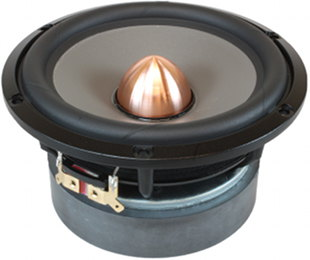open a bigger photo

recommended cabinet 1:
closed cabinet with 3,1 L volume
from 85/53 Hz (-3dB/-8dB)

recommended cabinet 2:
10,3 L volume bass reflex cabinet
with HP35 reflex tube, 11 cm long.
from 45/35 Hz (-3dB/-8dB).

## Excel W16NX003 (E0073-08)

order no. seas-E0073-08

EUR 1249,68excl. VAT: € 1077.31 / \$ 1271.23

13 cm bass driver with magnesium cone. The magnesium cone is treated by a monomolecular graphene layer. A pair of speakers is delivered (the price is for one pair).

• power handling (continuous/programme) = 180/300 W
• frequency range = 20-3000 Hz
• resonance frequency fs = 36 Hz
• impedance R = 8 Ohm
• sound pressure level SPL = 85,4 dB (2,83V; 1m)
• DC resistance Re = 6,4 Ohm
• force factor BL = 7,6 N/A
• voice coil inductance L = 0,12 mH
• effective piston radiating area Sd = 94 cm2
• effective mechanical mass incl. air load mms = 14,2 g
• equivalent volume of compliance Vas = 17 l
• total Q factor Qts = 0,33 (Qms=4,73, Qes=0,36)
• voice coil diameter = 39 mm
• maximum peak linear excursion vibration xlin = +/- 7 mm
• mounting diameter d = 126 mm
• overall diameter d = 146 mm
• mounting depth (not countersunk) t = 73,8 mm
PDF data sheet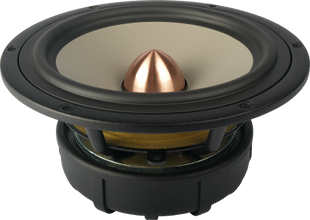open a bigger photo

recommended cabinet 1:
closed cabinet with 3 L volume
from 104/65 Hz (-3dB/-8dB)

recommended cabinet 2:
7,7 L volume bass reflex cabinet
with HP50 reflex tube, 22 cm long.
from 61/46 Hz (-3dB/-8dB).

## Excel W18EX001 (E0017-08S)

order no. seas-E0017-08S

EUR 350,92excl. VAT: € 302.52 / \$ 356.97

16 cm bass driver with magnesium cone.

• power handling (continuous/programme) = 100/250 W
• frequency range = 40-2500 Hz
• resonance frequency fs = 34 Hz
• impedance R = 8 Ohm
• sound pressure level SPL = 88 dB (2,83V; 1m)
• DC resistance Re = 6,1 Ohm
• force factor BL = 8,4 N/A
• voice coil inductance L = 0,65 mH
• effective piston radiating area Sd = 126 cm2
• effective mechanical mass incl. air load mms = 15,5 g
• equivalent volume of compliance Vas = 30 l
• total Q factor Qts = 0,26 (Qms=1,8, Qes=0,3)
• voice coil diameter = 39 mm
• maximum peak linear excursion vibration xlin = +/- 5 mm
• mounting diameter d = 145 mm
• overall diameter d = 176 mm
• mounting depth (not countersunk) t = 81 mm
PDF data sheet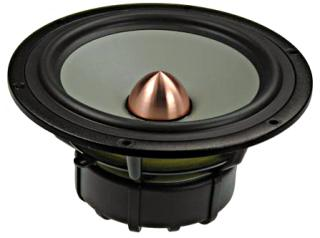recommended cabinet 1:
closed cabinet with 9,4 L volume
from 65/41 Hz (-3dB/-8dB)

recommended cabinet 2:
36 L volume bass reflex cabinet
with HP70 reflex tube, 21 cm long.
from 32/26 Hz (-3dB/-8dB).

## Excel W18E001 (E0018-08S)

order no. seas-E0018-08S

EUR 344,10excl. VAT: € 296.64 / \$ 350.03

16 cm bass driver with magnesium cone.

• power handling (continuous/programme) = 100/250 W
• frequency range = 40-2500 Hz
• resonance frequency fs = 34 Hz
• impedance R = 8 Ohm
• sound pressure level SPL = 86 dB (2,83V; 1m)
• DC resistance Re = 6,1 Ohm
• force factor BL = 6,6 N/A
• voice coil inductance L = 0,65 mH
• effective piston radiating area Sd = 126 cm2
• effective mechanical mass incl. air load mms = 15,5 g
• equivalent volume of compliance Vas = 30 l
• total Q factor Qts = 0,42 (Qms=2,91, Qes=0,49)
• voice coil diameter = 39 mm
• maximum peak linear excursion vibration xlin = +/- 5 mm
• mounting diameter d = 145 mm
• overall diameter d = 176 mm
• mounting depth (not countersunk) t = 81 mm
PDF data sheetrecommended cabinet 1:
closed cabinet with 4,4 L volume
from 94/59 Hz (-3dB/-8dB)

recommended cabinet 2:
14,6 L volume bass reflex cabinet
with HP50 reflex tube, 13 cm long.
from 50/39 Hz (-3dB/-8dB).

## Excel W18NX001 (E0042-08S)

order no. seas-E0042-08S

EUR 209,00excl. VAT: € 180.17 / \$ 212.60

16 cm bass driver with paper cone.

• power handling (continuous/programme) = 80/250 W
• frequency range = 30-3000 Hz
• resonance frequency fs = 40 Hz
• impedance R = 8 Ohm
• sound pressure level SPL = 87,5 dB (2,83V; 1m)
• DC resistance Re = 6,3 Ohm
• force factor BL = 7 N/A
• voice coil inductance L = 0,43 mH
• effective piston radiating area Sd = 126 cm2
• effective mechanical mass incl. air load mms = 13,7 g
• equivalent volume of compliance Vas = 24 l
• total Q factor Qts = 0,34 (Qms=2,08, Qes=0,41)
• voice coil diameter = 39 mm
• maximum peak linear excursion vibration xlin = +/- 7 mm
• mounting diameter d = 145,2 mm
• overall diameter d = 176 mm
• mounting depth (not countersunk) t = 81 mm
PDF data sheet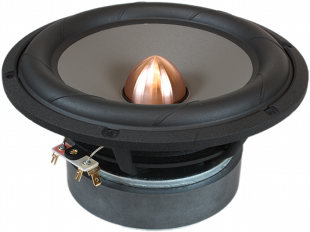open a bigger photo

recommended cabinet 1:
closed cabinet with 7,5 L volume
from 65/41 Hz (-3dB/-8dB)

recommended cabinet 2:
29 L volume bass reflex cabinet
with HP70 reflex tube, 28 cm long.
from 32/26 Hz (-3dB/-8dB).

## Excel W19NX001 (E0076-08)

order no. seas-E0076-08

EUR 1359,83excl. VAT: € 1172.27 / \$ 1383.28

16 cm bass driver with magnesium cone. The magnesium cone is treated by a monomolecular graphene layer. A pair of speakers is delivered (the price is for one pair).

• power handling (continuous/programme) = 200/360 W
• frequency range = 20-2000 Hz
• resonance frequency fs = 34 Hz
• impedance R = 8 Ohm
• sound pressure level SPL = 85,6 dB (2,83V; 1m)
• DC resistance Re = 6,2 Ohm
• force factor BL = 8,3 N/A
• voice coil inductance L = 0,23 mH
• effective piston radiating area Sd = 136 cm2
• effective mechanical mass incl. air load mms = 23,1 g
• equivalent volume of compliance Vas = 24 l
• total Q factor Qts = 0,41 (Qms=5,3, Qes=0,45)
• voice coil diameter = 39 mm
• maximum peak linear excursion vibration xlin = +/- 7 mm
• mounting diameter d = 153 mm
• overall diameter d = 176 mm
• mounting depth (not countersunk) t = 80,7 mm
PDF data sheetrecommended cabinet 1:
closed cabinet with 18 L volume
from 59/37 Hz (-3dB/-8dB)

recommended cabinet 2:
59 L volume bass reflex cabinet
with HP100 reflex tube, 36 cm long.
from 31/24 Hz (-3dB/-8dB).

## Excel W22EX001 (E0022-08S)

order no. seas-E0022-08S

EUR 390,89excl. VAT: € 336.97 / \$ 397.63

20 cm bass driver with magnesium cone.

• power handling (continuous/programme) = 120/300 W
• frequency range = 30-2000 Hz
• resonance frequency fs = 25 Hz
• impedance R = 8 Ohm
• sound pressure level SPL = 88 dB (2,83V; 1m)
• DC resistance Re = 6,1 Ohm
• force factor BL = 8,1 N/A
• voice coil inductance L = 0,61 mH
• effective piston radiating area Sd = 220 cm2
• effective mechanical mass incl. air load mms = 26,2 g
• equivalent volume of compliance Vas = 98 l
• total Q factor Qts = 0,34 (Qms=2,14, Qes=0,41)
• voice coil diameter = 39 mm
• maximum peak linear excursion vibration xlin = +/- 5 mm
• mounting diameter d = 186,4 mm
• overall diameter d = 220,6 mm
• mounting depth (not countersunk) t = 93 mm
PDF data sheet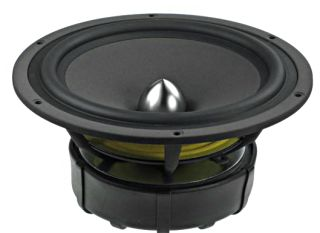recommended cabinet 1:
closed cabinet with 9,9 L volume
from 88/55 Hz (-3dB/-8dB)

recommended cabinet 2:
29 L volume bass reflex cabinet
with HP70 reflex tube, 14 cm long.
from 49/37 Hz (-3dB/-8dB).

## Excel W22NY001 (E0045-08S)

order no. seas-E0045-08S

EUR 334,35excl. VAT: € 288.23 / \$ 340.11

20 cm bass driver with paper cone.

• power handling (continuous/programme) = 90/250 W
• frequency range = 30-3000 Hz
• resonance frequency fs = 33 Hz
• impedance R = 8 Ohm
• sound pressure level SPL = 90 dB (2,83V; 1m)
• DC resistance Re = 6,1 Ohm
• force factor BL = 8,8 N/A
• voice coil inductance L = 0,61 mH
• effective piston radiating area Sd = 220 cm2
• effective mechanical mass incl. air load mms = 20 g
• equivalent volume of compliance Vas = 72 l
• total Q factor Qts = 0,3 (Qms=1,73, Qes=0,36)
• voice coil diameter = 39 mm
• maximum peak linear excursion vibration xlin = +/- 7 mm
• mounting diameter d = 186,4 mm
• overall diameter d = 220,6 mm
• mounting depth (not countersunk) t = 100 mm
PDF data sheet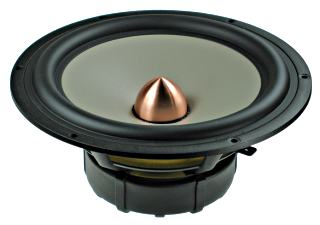recommended cabinet 1:
closed cabinet with 32 L volume
from 46/29 Hz (-3dB/-8dB)

recommended cabinet 2:
108 L volume bass reflex cabinet
with HP100 reflex tube, 32 cm long.
from 24/19 Hz (-3dB/-8dB).

## Excel W26FX001 (E0026-08S)

order no. seas-E0026-08S

EUR 523,46excl. VAT: € 451.26 / \$ 532.49

25 cm bass driver with magnesium cone.

• power handling (continuous/programme) = 150/400 W
• frequency range = 20-1000 Hz
• resonance frequency fs = 20 Hz
• impedance R = 8 Ohm
• sound pressure level SPL = 87 dB (2,83V; 1m)
• DC resistance Re = 6,3 Ohm
• force factor BL = 10,7 N/A
• voice coil inductance L = 1,43 mH
• effective piston radiating area Sd = 330 cm2
• effective mechanical mass incl. air load mms = 56,3 g
• equivalent volume of compliance Vas = 162 l
• total Q factor Qts = 0,35 (Qms=2,34, Qes=0,41)
• voice coil diameter = 51 mm
• maximum peak linear excursion vibration xlin = +/- 7 mm
• mounting diameter d = 232,2 mm
• overall diameter d = 269 mm
• mounting depth (not countersunk) t = 109,5 mm
PDF data sheet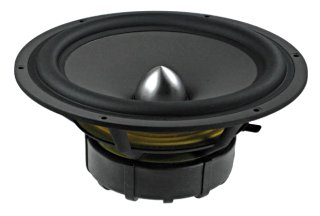recommended cabinet 1:
closed cabinet with 24 L volume
from 62/39 Hz (-3dB/-8dB)

recommended cabinet 2:
89 L volume bass reflex cabinet
with HP100 reflex tube, 18 cm long.
from 31/25 Hz (-3dB/-8dB).

## Excel W26FX002 (E0046-08S)

order no. seas-E0046-08S

EUR 457,18excl. VAT: € 394.12 / \$ 465.06

25 cm bass driver with paper cone.

• power handling (continuous/programme) = 100/300 W
• frequency range = 30-1000 Hz
• resonance frequency fs = 31 Hz
• impedance R = 8 Ohm
• sound pressure level SPL = 89 dB (2,83V; 1m)
• DC resistance Re = 6,2 Ohm
• force factor BL = 10,9 N/A
• voice coil inductance L = 0,81 mH
• effective piston radiating area Sd = 330 cm2
• effective mechanical mass incl. air load mms = 42,4 g
• equivalent volume of compliance Vas = 87 l
• total Q factor Qts = 0,4 (Qms=2,55, Qes=0,47)
• voice coil diameter = 51 mm
• maximum peak linear excursion vibration xlin = +/- 7 mm
• mounting diameter d = 232,2 mm
• overall diameter d = 269 mm
• mounting depth (not countersunk) t = 109,5 mm
PDF data sheet

## Coaxial speakeropen a bigger photo

recommended cabinet 1:
closed cabinet with 8,3 L volume
from 59/37 Hz (-3dB/-8dB)

recommended cabinet 2:
34 L volume bass reflex cabinet
with HP50 reflex tube, 14 cm long.
from 27/23 Hz (-3dB/-8dB).

## Excel C16N001/F (E0051-04/06S)

order no. seas-E0051-04-06S

EUR 320,71excl. VAT: € 276.47 / \$ 326.24

13 cm coax driver with magnesium cone.

• power handling (continuous/programme) = 80/250 W
• frequency range = 30-25000 Hz
• resonance frequency fs = 37 Hz
• impedance R = 4 Ohm
• sound pressure level SPL = 85 dB (2,83V; 1m)
• DC resistance Re = 3,2 Ohm
• force factor BL = 4,4 N/A
• voice coil inductance L = 0,27 mH
• effective piston radiating area Sd = 94 cm2
• effective mechanical mass incl. air load mms = 14,6 g
• equivalent volume of compliance Vas = 15 l
• total Q factor Qts = 0,49 (Qms=3,24, Qes=0,58)
• voice coil diameter = 39 mm
• maximum peak linear excursion vibration xlin = +/- 7 mm
• mounting diameter d = 126 mm
• overall diameter d = 146 mm
• mounting depth (not countersunk) t = 85,5 mm
PDF data sheet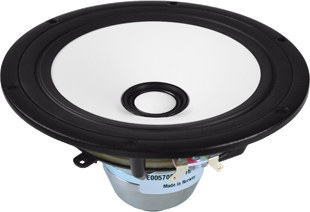open a bigger photo

recommended cabinet 1:
closed cabinet with 3,4 L volume
from 127/80 Hz (-3dB/-8dB)

recommended cabinet 2:
13,7 L volume bass reflex cabinet
with HP70 reflex tube, 13 cm long.
from 58/50 Hz (-3dB/-8dB).

## Excel C18EN002/A (E0060-08/06)

order no. seas-E0060-08-06S

EUR 574,15excl. VAT: € 494.96 / \$ 584.05

17 cm coax driver with magnesium cone.

• power handling (continuous/programme) = 100/250 W
• frequency range = 150-30000 Hz
• resonance frequency fs = 85 Hz
• impedance R = 8 Ohm
• sound pressure level SPL = 87 dB (2,83V; 1m)
• DC resistance Re = 6,3 Ohm
• force factor BL = 8,8 N/A
• voice coil inductance L = 0,46 mH
• effective piston radiating area Sd = 131 cm2
• effective mechanical mass incl. air load mms = 15,3 g
• equivalent volume of compliance Vas = 5 l
• total Q factor Qts = 0,54 (Qms=1,99, Qes=0,74)
• voice coil diameter = 39 mm
• maximum peak linear excursion vibration xlin = +/- 3 mm
• mounting diameter d = 176 mm
• overall diameter d = 176 mm
• mounting depth (not countersunk) t = 93 mm
PDF data sheet

## no longer sold## Excel M15CH001 (E0016-08S)

order no. not-available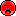- not available

cm bass-midrange driver with high quality cone.

• power handling (continuous/programme) = 100/300 W
• frequency range = 150-3000 Hz
• resonance frequency fs = 54 Hz
• impedance R = 8 Ohm
• sound pressure level SPL = 90 dB (2,83V; 1m)
• DC resistance Re = 5,7 Ohm
• force factor BL = 5,5 N/A
• voice coil inductance L = 0,31 mH
• effective piston radiating area Sd = 75 cm2
• effective mechanical mass incl. air load mms = 5,8 g
• equivalent volume of compliance Vas = 10,9 l
• total Q factor Qts = 0,3 (Qms=1,1, Qes=0,41)
• voice coil diameter = 26 mm
• maximum peak linear excursion vibration xlin = +/- 1 mm
PDF data sheet

## Excel T29MF001 (E0047-04) Magnum

order no. not-available- not available

26 mm dome tweeter with magnesium dome. The speaker has a 6 mm solid metal front plate. A unique magnet system based on 6 radially magnetized Neodymium magnet blocks is used.

• power handling (continuous/programme) = 90/200 W
• frequency range = 2000-25000 Hz
• resonance frequency fs = 500 Hz
• impedance R = 4 Ohm
• sound pressure level SPL = 92 dB (2,83V; 1m)
• DC resistance Re = 3,5 Ohm
• force factor BL = 3 N/A
• voice coil inductance L = 0,03 mH
• effective piston radiating area Sd = 8 cm2
• effective mechanical mass incl. air load mms = 0,35 g
• voice coil diameter = 26 mm
• maximum peak linear excursion vibration xlin = +/- 0,7 mm
• mounting diameter d = 85 mm
• overall diameter d = 110 mm
• mounting depth (not countersunk) t = 40 mm
PDF data sheet## Excel W11 CY001

order no. not-available- not available

10 cm bass driver with paper cone. The cone is equipped with a copper phase plug.

• power handling (continuous/programme) = 75/200 W
• resonance frequency fs = 65 Hz
• impedance R = 8 Ohm
• sound pressure level SPL = 86 dB (2,83V; 1m)
• DC resistance Re = 5,7 Ohm
• force factor BL = 5,6 N/A
• voice coil inductance L = 0,32 mH
• effective piston radiating area Sd = 50 cm2
• effective mechanical mass incl. air load mms = 5,5 g
• total Q factor Qts = 0,34 (Qms=1,8, Qes=0,42)
• voice coil diameter = 26 mm
• maximum peak linear excursion vibration xlin = +/- 3 mm
• mounting diameter d = 96 mm
• overall diameter d = 109,4 mm
• mounting depth (not countersunk) t = 55,1 mmrecommended cabinet 1:
closed cabinet with 0,86 L volume
from 118/75 Hz (-3dB/-8dB)

recommended cabinet 2:
2,6 L volume bass reflex cabinet
with HP35 reflex tube, 25 cm long.
from 65/50 Hz (-3dB/-8dB).

## Excel W11 CY002

order no. not-available- not available

10 cm midrange driver with magnesium cone. The cone is equipped with a copper phase plug.

• power handling (continuous/programme) = 75/200 W
• resonance frequency fs = 46 Hz
• impedance R = 8 Ohm
• sound pressure level SPL = 85 dB (2,83V; 1m)
• DC resistance Re = 5,6 Ohm
• force factor BL = 5,6 N/A
• voice coil inductance L = 0,32 mH
• effective piston radiating area Sd = 50 cm2
• effective mechanical mass incl. air load mms = 7,2 g
• equivalent volume of compliance Vas = 5,8 l
• total Q factor Qts = 0,31 (Qms=1,9, Qes=0,37)
• voice coil diameter = 26 mm
• maximum peak linear excursion vibration xlin = +/- 3 mm
• mounting diameter d = 96 mm
• overall diameter d = 109,4 mm
• mounting depth (not countersunk) t = 55,1 mmrecommended cabinet 1:
closed cabinet with 2,1 L volume
from 98/62 Hz (-3dB/-8dB)

recommended cabinet 2:
7,1 L volume bass reflex cabinet
with HP35 reflex tube, 12 cm long.
from 51/40 Hz (-3dB/-8dB).

## Excel W14 CY001

order no. not-available- not available

12 cm bass driver with magnesium cone.

• power handling (continuous/programme) = 75/120 W
• resonance frequency fs = 43 Hz
• impedance R = 8 Ohm
• sound pressure level SPL = 87 dB (2,83V; 1m)
• DC resistance Re = 5,4 Ohm
• force factor BL = 5,8 N/A
• voice coil inductance L = 0,37 mH
• effective piston radiating area Sd = 75 cm2
• effective mechanical mass incl. air load mms = 9,6 g
• equivalent volume of compliance Vas = 10,6 l
• total Q factor Qts = 0,35 (Qms=1,8, Qes=0,44)
• voice coil diameter = 26 mm
• maximum peak linear excursion vibration xlin = +/- 4 mm
• mounting diameter d = 113 mm
• overall diameter d = 133,2 mm
• mounting depth (not countersunk) t = 65,3 mm
PDF data sheetrecommended cabinet 1:
closed cabinet with 4,7 L volume
from 85/54 Hz (-3dB/-8dB)

recommended cabinet 2:
14,7 L volume bass reflex cabinet
with HP50 reflex tube, 16 cm long.
from 46/35 Hz (-3dB/-8dB).

## Excel W17 E002

order no. not-available- not available

16 cm bass driver with magnesium cone. The cone is equipped with a copper phase plug.

• power handling (continuous/programme) = 100/250 W
• resonance frequency fs = 34 Hz
• impedance R = 8 Ohm
• sound pressure level SPL = 87 dB (2,83V; 1m)
• DC resistance Re = 6,4 Ohm
• force factor BL = 7,8 N/A
• voice coil inductance L = 0,4 mH
• effective piston radiating area Sd = 126 cm2
• effective mechanical mass incl. air load mms = 15,5 g
• equivalent volume of compliance Vas = 29,5 l
• total Q factor Qts = 0,32 (Qms=2,4, Qes=0,37)
• voice coil diameter = 39 mm
• maximum peak linear excursion vibration xlin = +/- 4 mm
• mounting diameter d = 146 mm
• overall diameter d = 170,4 mm
• mounting depth (not countersunk) t = 67,7 mmrecommended cabinet 1:
closed cabinet with 4,4 L volume
from 92/58 Hz (-3dB/-8dB)

recommended cabinet 2:
13,7 L volume bass reflex cabinet
with HP50 reflex tube, 15 cm long.
from 50/39 Hz (-3dB/-8dB).

## Excel W17 EX001

order no. not-available- not available

16 cm bass driver with glass fibre cone. The cone is equipped with a copper phase plug.

• power handling (continuous/programme) = 100/250 W
• resonance frequency fs = 37 Hz
• impedance R = 8 Ohm
• sound pressure level SPL = 89 dB (2,83V; 1m)
• DC resistance Re = 6,1 Ohm
• force factor BL = 8,5 N/A
• voice coil inductance L = 0,32 mH
• effective piston radiating area Sd = 130 cm2
• effective mechanical mass incl. air load mms = 15 g
• equivalent volume of compliance Vas = 27,4 l
• total Q factor Qts = 0,32 (Qms=3,38, Qes=0,35)
• voice coil diameter = 39 mm
• maximum peak linear excursion vibration xlin = +/- 4 mm
• mounting diameter d = 145,3 mm
• overall diameter d = 170,4 mm
• mounting depth (not countersunk) t = 67,7 mmrecommended cabinet 1:
closed cabinet with 3 L volume
from 104/65 Hz (-3dB/-8dB)

recommended cabinet 2:
7,5 L volume bass reflex cabinet
with HP50 reflex tube, 23 cm long.
from 61/46 Hz (-3dB/-8dB).

## Excel W17 EX002

order no. not-available- not available

16 cm bass driver with magnesium cone. The cone is equipped with a copper phase plug.

• power handling (continuous/programme) = 100/250 W
• resonance frequency fs = 34 Hz
• impedance R = 8 Ohm
• sound pressure level SPL = 88 dB (2,83V; 1m)
• DC resistance Re = 6,4 Ohm
• force factor BL = 8,6 N/A
• voice coil inductance L = 0,4 mH
• effective piston radiating area Sd = 126 cm2
• effective mechanical mass incl. air load mms = 15,5 g
• equivalent volume of compliance Vas = 29,5 l
• total Q factor Qts = 0,26 (Qms=2,1, Qes=0,3)
• voice coil diameter = 39 mm
• maximum peak linear excursion vibration xlin = +/- 4 mm
• mounting diameter d = 146 mm
• overall diameter d = 170,4 mm
• mounting depth (not countersunk) t = 67,7 mmrecommended cabinet 1:
closed cabinet with 19 L volume
from 61/38 Hz (-3dB/-8dB)

recommended cabinet 2:
71 L volume bass reflex cabinet
with HP100 reflex tube, 26 cm long.
from 30/24 Hz (-3dB/-8dB).

## Excel W21 EX001

order no. not-available- not available

20 cm bass driver with high quality cone. The cone is equipped with a copper phase plug.

• power handling (continuous/programme) = 120/300 W
• resonance frequency fs = 31 Hz
• impedance R = 8 Ohm
• sound pressure level SPL = 91 dB (2,83V; 1m)
• DC resistance Re = 6,4 Ohm
• force factor BL = 8,5 N/A
• voice coil inductance L = 0,32 mH
• effective piston radiating area Sd = 220 cm2
• effective mechanical mass incl. air load mms = 25 g
• equivalent volume of compliance Vas = 64 l
• total Q factor Qts = 0,41 (Qms=2,73, Qes=0,48)
• voice coil diameter = 39 mm
• maximum peak linear excursion vibration xlin = +/- 6 mm
• mounting diameter d = 186,8 mm
• overall diameter d = 215,4 mm
• mounting depth (not countersunk) t = 75,3 mmrecommended cabinet 1:
closed cabinet with 30 L volume
from 50/31 Hz (-3dB/-8dB)

recommended cabinet 2:
120 L volume bass reflex cabinet
with HP100 reflex tube, 22 cm long.
from 23/20 Hz (-3dB/-8dB).

## Excel W21 E002

order no. not-available- not available

20 cm bass driver with magnesium cone. The cone is equipped with a copper phase plug.

• power handling (continuous/programme) = 120/300 W
• resonance frequency fs = 29 Hz
• impedance R = 8 Ohm
• sound pressure level SPL = 89 dB (2,83V; 1m)
• DC resistance Re = 6,4 Ohm
• force factor BL = 7,9 N/A
• voice coil inductance L = 0,4 mH
• effective piston radiating area Sd = 220 cm2
• effective mechanical mass incl. air load mms = 28 g
• equivalent volume of compliance Vas = 69 l
• total Q factor Qts = 0,47 (Qms=3,2, Qes=0,55)
• voice coil diameter = 39 mm
• maximum peak linear excursion vibration xlin = +/- 4 mm
• mounting diameter d = 187 mm
• overall diameter d = 215,4 mm
• mounting depth (not countersunk) t = 80,3 mmrecommended cabinet 1:
closed cabinet with 46 L volume
from 49/31 Hz (-3dB/-8dB)

recommended cabinet 2:
188 L volume bass reflex cabinet
with HP100 reflex tube, 12 cm long.
from 22/19 Hz (-3dB/-8dB).

## Excel W21 E002-4

order no. not-available- not available

20 cm bass driver with magnesium cone. The cone is equipped with a copper phase plug.

• power handling (continuous/programme) = 120/300 W
• resonance frequency fs = 33 Hz
• impedance R = 4 Ohm
• sound pressure level SPL = 89 dB (2,83V; 1m)
• DC resistance Re = 2,9 Ohm
• force factor BL = 5,4 N/A
• voice coil inductance L = 0,24 mH
• effective piston radiating area Sd = 220 cm2
• effective mechanical mass incl. air load mms = 26 g
• equivalent volume of compliance Vas = 69 l
• total Q factor Qts = 0,51 (Qms=4,42, Qes=0,58)
• voice coil diameter = 39 mm
• maximum peak linear excursion vibration xlin = +/- 4 mm
• mounting diameter d = 187 mm
• overall diameter d = 215,4 mm
• mounting depth (not countersunk) t = 80,3 mmrecommended cabinet 1:
closed cabinet with 14,6 L volume
from 62/39 Hz (-3dB/-8dB)

recommended cabinet 2:
50 L volume bass reflex cabinet
with HP70 reflex tube, 16 cm long.
from 32/25 Hz (-3dB/-8dB).

## Excel W21 EX002

order no. not-available- not available

20 cm bass driver with magnesium cone. The cone is equipped with a copper phase plug.

• power handling (continuous/programme) = 120/300 W
• resonance frequency fs = 28 Hz
• impedance R = 8 Ohm
• sound pressure level SPL = 91 dB (2,83V; 1m)
• DC resistance Re = 6,4 Ohm
• force factor BL = 9,2 N/A
• voice coil inductance L = 0,5 mH
• effective piston radiating area Sd = 220 cm2
• effective mechanical mass incl. air load mms = 29 g
• equivalent volume of compliance Vas = 69 l
• total Q factor Qts = 0,36 (Qms=2,7, Qes=0,41)
• voice coil diameter = 39 mm
• maximum peak linear excursion vibration xlin = +/- 5 mm
• mounting diameter d = 187 mm
• overall diameter d = 215,4 mm
• mounting depth (not countersunk) t = 80,3 mm

The cabinet volume calculations are executed considering an external resistance of 0.3 Ohm. We are using, if no other data is known or available, the information supplied by the manufacturer.

Please note: the frequency response doesn't say much about the cut-off frequency inside the cabinet! Therefore, we mostly give you 2 examples with dimensioning considerations and an indication, how low the loudspeaker will reproduce.

Home